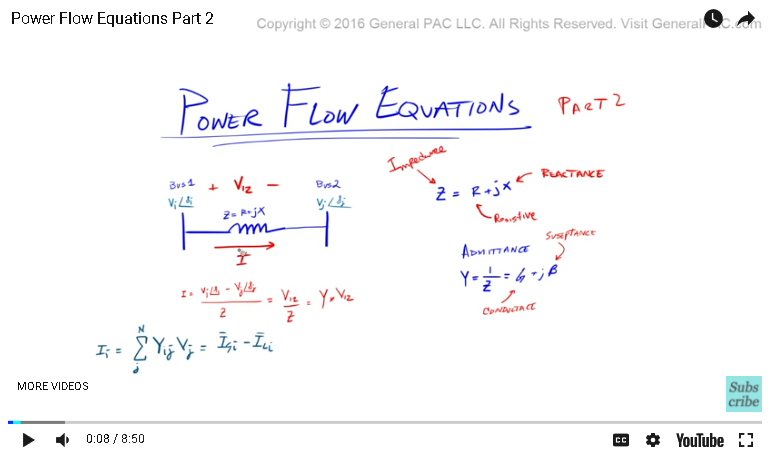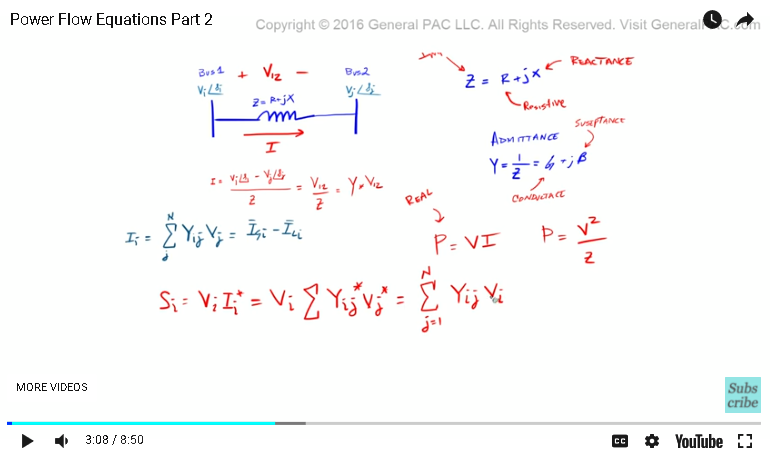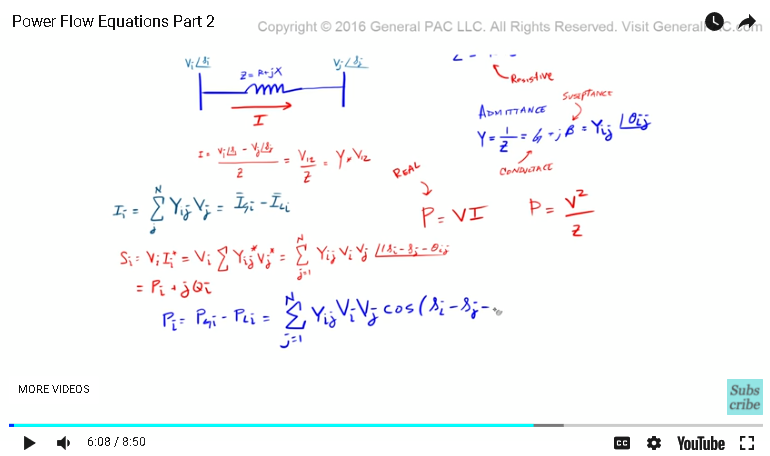This video was brought to you by GeneralPAC.com, making power systems Intuitive, Open and Free for Everyone, Everywhere. Consider subscribing and supporting through patreon.com/GeneralPAC. This is a mechanism for you to support us financially so we can continue making high quality power system video tutorials. Our corporate sponsor for this topic is AllumiaX.com from Seattle, Washington. Contact them for industrial and commercial power system studies.

A tutorial on power flow equations, part twoOkay, so in part one we talked about setting up a scenario. We talked about voltages and current on a particular bus, the impedance, the admittances and how to define current for a multi bus system. In part two we'll talk about power. Now when we think of power what are some of the equations that come to mind? So one equation that comes to my mind for example is that power is equal to voltage times current and power is also equal to the voltage squared over the impedance or the resistance. Let's put that over here. Obviously this is real power. This term here, the P term, is the real power. In a power system what we have is we have a real power and a reactive power and both of those combined is called the apparent power. So the apparent power of the ith bus, and we'll talk about that a little more about what this particular term means.

So, we're saying that this is voltage and this term is basically that term there and so the admittance times the voltage, all we're saying is the admittance is just the one over the impedance, and we're multiplying by the voltage, the voltage is just voltage is equal to V over C. We all know that by Ohm's law if you take the voltage over an impedance that is equal to the current. So all we're doing is we're redefining what this current is, and we're putting it in this form. So nothing new here. That is equal to the sum of the terms, and we'll start from J is equal to 1 to the nth bus. Multiply that by the admittance Y, I, J, nothing new there, times the voltage of the ith bus. Remember these two terms, multiply that together and that's going to equal the current. Then we multiply that by the voltage of the jth bus. Then we have to now take account of the angles of all of these terms.So now we'll put this big angle sign here and so remember the voltage on bus one has this tilde I. The voltage on bus 2 for example had tilde J and then we had this. So the admittance is going be equal to Y, I, J and that is going to have an angle of theta I, J. So that's what the admittance is. We'll talk about this a little bit more later. The angle of apparent power is equal to the magnitude of admittance times the magnitude of the voltage at the ith bus times the magnitude of the voltage at the jth bus and then now let's account for the angles. So the angles are going to equal, now we have the ith angle, which is the angle of this voltage here, minus the jth angle, which is the angle of the voltage on the jth bus minus the angle of theta I, J, which is the angle of the admittance value.So the next part is, like we said before, apparent power can be broken up into real and reactive components and so the real component ... Before we talk about the real component, let's make this smaller, so SI is equal to the PI plus JQI. So here it is. So the apparent power is equal to the real power plus this reactive power, so these two components. The next is, well let's break these two terms, so PI, so the real power of the ith bus is equal to the power of the ith bus that's generated minus the power of the ith bus of the load, the generated minus the load, that is equal to the sum, J is equal to 1 to the nth bus, multiply that by YIJ, similar to that, multiply that by VI, multiply that by VJ and then we have this cosign term. So we'll throw in this cosign and we'll say the cosign of the angle of I, minus the angle of J, minus the theta of IJ. So this cosign term is placed here and then let's do this term right here, the reactive power.

So the reactive power, which is QI that is equal to QGI. So the total generated reactive power of the ith bus minus QLI, the total load reactive power of the ith bus, that is equal to the sum of J is equal to 1 to the nth bus. The same thing here. Not doing anything special but here is where it's different. Now we're taking this sign term. Sign of the angle of the voltage on the ith bus minus the angle of the voltage on the jth bus minus theta IJ. Let's make this smaller. It's really important to relate all three powers. Now we know this very well. I'll just review it quickly now.

So how do you relate these three terms? Well, the way to relate these three terms is by the power triangle. This is called the power triangle. What we're saying is that we have this angle, this angle of this triangle. It's a right triangle by the way. The hypotenuse, which is the longer piece here, that is called the apparent power. Then the adjacent, which is this part here, that is called the real power and then the opposite term, which is this part here, that is called the reactive power. So S is equal to P plus JQ. Now if we knew what S is and we wanted to solve for P then P is equal to S, what we know already, times that by the cosign of this angle.

Similarly Q, which is this opposite term here, is equal to S times the sign of this angle, this angle here. So it is very easy to relate all of these three powers and that's essentially what we're doing here. We know what S is, we know what all of these angles are, now we're just breaking up this apparent power into the real component and the reactive component.

#### Greetings from the GeneralPAC Team!

We make high-quality Power Systems Video Tutorials on complex topics that are free and open to everyone!  Thank you so much for supporting us through Patreon so we can continue doing good and valuable work.

What is Patreon and why do we use it?

Patreon is a fantastic portal that allows our fans and community to make monthly contribution (like Netflix subscription) so we can continue creating high-quality power systems video tutorials. In return, you get access to incredible perks like voting on future topics, getting your questions answered, access to VIP Q/A webinars with the creators of GeneralPAC, and much more! We THANK YOU for supporting us

Why do we need your support?

An incredible amount of time and effort is needed to develop high-quality video tutorials. Each video (Part 1 for example) takes approximately 10 hours to complete which includes learning the concept ourselves, brainstorming creative ways to teach and explain the concepts, writing the script, audio recording, video recording, and editing. It's no wonder why Hundreds-of-Thousands of people have watched, liked, subscribed, and left positive comments on Youtube channel. Your support truly makes all the difference.